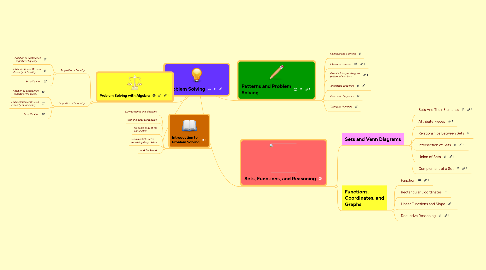# Problem Solving

Get Started. It's FreeProblem Solving## 3. Problem Solving with Algebra

### 3.1. Properties of Equality

3.1.1. Addition or Subtraction Property of Equality

3.1.2. Multiplication or Division Property of Equality

3.1.3. Simplification

### 3.2. Properties of Inequality

3.2.1. Addition or Subtraction Property of Inequality

3.2.2. Multiplication or Division Property of Inequality

3.2.3. Simplification

## 4. Sets, Functions, and Reasoning

### 4.1. Sets and Venn Diagrams

4.1.1. Sets And Their Elements

4.1.2. Attribute Pieces

4.1.3. Relationships Between Sets

4.1.4. Intersection of Sets

4.1.5. Union of Sets

4.1.6. Complement of a Set

### 4.2. Functions, Coordinates, and Graphs

4.2.1. Function

4.2.2. Rectangular Coordinates

4.2.3. Linear Functions and Slope

4.2.4. Deductive Reasoning